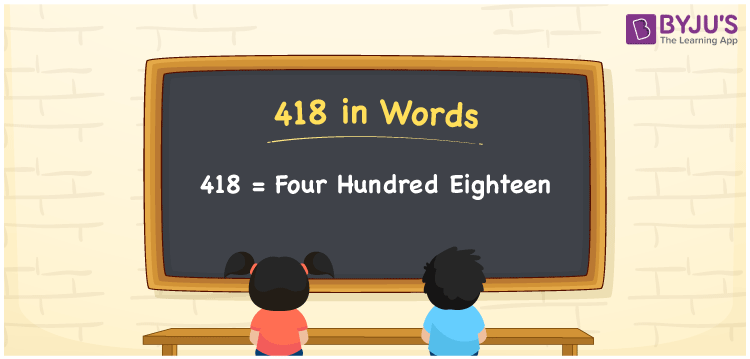# 418 in words

418 in words is written as Four Hundred and Eighteen. 418 represents the count or value. The article on Counting Numbers can give you an idea about count or counting. The number 418 is a 3 digit number that is used in expressions related to money, days, distance, length, weight and so on. Let us consider an example for 418. “The distance between Point A and Point B is Four Hundred and Eighteen kilometers.”

 418 in words Four Hundred and Eighteen Four Hundred and Eighteen in Numbers 418

## 418 in English Words## How to Write 418 in Words?

We can convert 418 to words using a place value chart. The number 418 has 3 digits, so let’s make a chart that shows the place value up to 3 digits.

 Hundreds Tens Ones 4 1 8

Thus, we can write the expanded form as:

4 × Hundred + 1 × Ten + 8 × One

= 4 × 100 + 1 × 10 + 8 × 1

= 418

= Four Hundred and Eighteen.

418 is the natural number that is succeeded by 417 and preceded by 419.

418 in words – Four Hundred and Eighteen.

Is 418 an odd number? – No.

Is 418 an even number? – Yes.

Is 418 a perfect square number? – No.

Is 418 a perfect cube number? – No.

Is 418 a prime number? – No.

Is 418 a composite number? – Yes.

## Solved Example

1. Write the number 418 in expanded form

Solution: 4 × 100 + 1 × 10 + 8 × 1

We can write 418 = 400 + 10 + 8

= 4 × 100 + 1 × 10 + 8 × 1.

## Frequently Asked Questions on 418 in words

Q1

### How to write the number 418 in words?

418 in words is written as Four Hundred and Eighteen.
Q2

### Is 418 divisible by 3?

No. 418 is not divisible by 3.
Q3

### Is 418 a perfect square number?

No. 418 is not a perfect square number.